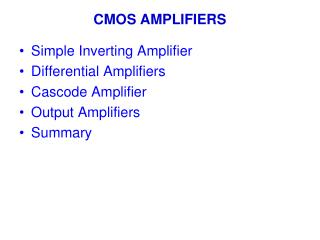Download PresentationCMOS AMPLIFIERS

CMOS AMPLIFIERS - PowerPoint PPT Presentation

CMOS AMPLIFIERS. Simple Inverting Amplifier Differential Amplifiers Cascode Amplifier Output Amplifiers Summary. Simple Inverting Amplifiers. Small Signal Characteristics. Inverter with diode connection load. How do you get better matching?. High gain inverters.I am the owner, or an agent authorized to act on behalf of the owner, of the copyrighted work described.
Download PresentationCMOS AMPLIFIERS

Download Policy: Content on the Website is provided to you AS IS for your information and personal use and may not be sold / licensed / shared on other websites without getting consent from its author.While downloading, if for some reason you are not able to download a presentation, the publisher may have deleted the file from their server.

- - - - - - - - - - - - - - - - - - - - - - - - - - E N D - - - - - - - - - - - - - - - - - - - - - - - - - -
Presentation Transcript
1. CMOS AMPLIFIERS • Simple Inverting Amplifier • Differential Amplifiers • Cascode Amplifier • Output Amplifiers • Summary

2. Simple Inverting Amplifiers

3. Small Signal Characteristics Inverter with diode connection load How do you get better matching?

4. High gain inverters

5. Current source load or push-pull • Refer to book for large signal analysis • Must match quiescent currents in PMOS and NMOS transistors • Wider output swing, especially push-pull • Much higher gain (at DC), but much lower -3dB frequency (vs diode load) • About the same GB • Very power dependent

6. Small signal High gain! Especially at low power.

7. Key to analysis by hand: • Use level 1 or 3 model equations • Use KCL/KVL

8. Dependence of Gain upon Bias Current

9. Transfer function of a system input u output y System

10. When u(s) = 0, y(s) satisfies: These dynamics are the characteristic dynamics of the system. The roots of the coefficient polynomial are the poles of the system. When y(s) = 0, u(s) satisfies: These dynamics are the zero dynamics of the system. The roots of the coefficient polynomial are the zeros of the system.

11. Frequency Response of CMOS Inverters

12. Poles of CMOS Inverters Let vin = 0, x = 0, VDD = 0, VSS = 0. CGS1, CGS2, CBS1, CBS2 are all short y CGD1, CGD2, CBD1, CBD2, CL in parallel C’L = Ctotal = CGD1+ CGD2+ CBD1+ CBD2+ CL

13. Total conductance from y to ground: go = gds1 + gds2 KCL at node y: Therefore system pole is:

14. Zeros of CMOS Inverters Let vin = x = u, VDD = 0, VSS = 0. CGD1, CGD2, are in parallel, CBD1, CBD2, CL are all short gds1, gds2 also short No current in them KCL: Zero is:

15. Zeros of CMOS CS Load Amp Let vin = u, X=0, VDD = 0, VSS = 0. CGS2, CGD2, CBD1, CBD2, CL are all short gds1, gds2 also short No current in them KCL: Zero is:

16. Input output transfer function When s=jw0, A(0)  When w∞, A(s)

17. -3dB frequency of closed loop =b*GB |A0 | =gm/go Acl=1/b 0 dB |p1|= g0/CL’ Unity gain frequency =|A0p1| =GB =gm/CL’ |z1| =gm/Cgd =GB*CL’/Cgd

18. Unity gain feedback A(s) Closed-loop zero: z1

19. If a step input is given, the output response is By the final value theorem: By the initial value theorem:

20. -1 Final settling determined by A0  need high gain Settling speed determined by A0p1=GB=UGF,  need high gain bandwidth product

21. Gain bandwidth product C’L = Ctotal = CGD1+ CGD2+ CBD1+ CBD2+ CL When CL≈ C’L, W↑GB↑, but it saturates, when

22. Note: If VEB1 and VEB2 are fixed, W1/L1 and W2/L2 must be adjusted proportionally, and they are proportional to DC power.

23. Therefore: P is proportional to W1, W2 CL constant, but C(W1,W2) proportional to W1, W2 When C(W1, W2) << CL, GB proportional to P When C(W1,W2)CL or >CL, GB saturates

24. GB Linear increase region P

25. For given current or power (current source load) Initially, as W1 increased, GB increases But GB will reach a max, and then drop as W1 increases

26. NOISE IN MOS INVERTERS

27. To minimize: • L2 >>L1 • En1 small

28. For thermal noise

29. Noise in Push-Pull current source load Inverter

30. Differential Input, single-ended output single stage Amplifier N-Channel vin- vin+

31. P-channel

32. Large Signal Eq. in a N-channel Differential pair =0.5b1(VGS1-VT)2 =(2ID1/b1)0.5 iD1=0, when iD2=ISS and VGS2=VT+(2ISS/b)0.5

33. Solving for iD1 and iD2 iD1=iD2=ISS/2 VON1=VON2=(ISS/b)0.5

34. N-Channel Input Pair Differential Amplifier C.M. Load Simple current reference C.M. Bias

35. Voltage transfer curve

36. P-Channel Input Pair Differential Amplifier

37. Voltage transfer curve

38. INPUT COMMON MODE RANGE VG1=VG2=ViCM VSDSAT1=VSDSAT2 =VON VD1=VD3= VSS+VT3+VON VG1min=VD1-|VT1| VG1max=VDD- VSD5SAT-|VT1|-VON

39. Output Range Vomin=Vss+Von4 Vomax=Vicm –|VT2| So what’s the vo range What’s for the N-ch circuit.

40. Common Mode Equivalent Circuit, with perfect match iC1=VIC/(1/gm1 +2rds5) ro1≈1/gm3 ACM≈ 1/ 2rds5gm3 iC1

41. If not perfectly matched io=aiIC a is a fraction go1≈ gds4 + gds2/2Av5 ≈ gds4 iC1 ACM ≈ agm5 / 2gds4 CMRR=Av/ACM= gm1/agm5

42. Formal detailed analysis

43. SLEW RATE: the limit of the rate of change of the output voltage C’Ldvo/dt=i4-i2 Max |CLdvo/dt|=ISS ISS ISS Slew Rate = ISS/C’L 0 ISS Output swing: Vosw GB frequency: fGB vo(t)=Voswsin(2pfGBt) Max dvo/dt =Vosw2pfGB To avoid slewing: ISS > C’L Vosw2pfGB

44. Parasitic Capacitances CT: common mode only CM: mirror cap = Cdg1 + Cdb1 + Cgs3 + Cgs4 + Cdb3 COUT = output cap = Cbd4 + Cbd2 + Cgd2 + CL